# Tau (an unofficial brilliant wiki made by me)

$\large\tau$ is defined as $2\pi$ and if you don't know what $\pi$ is, its not the sweet treat, I can assure you.

$\pi$ is defined as the ratio of the circumference of a circle to its diameter. This means - $\pi = \dfrac{\text{circumference}}{\text{diameter}}$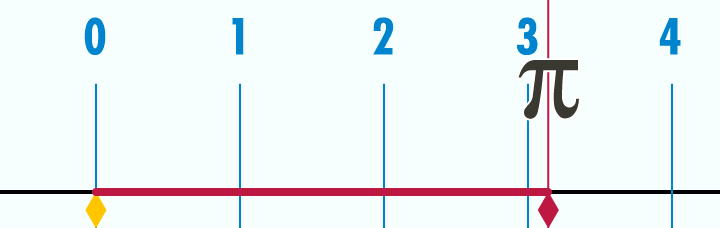$\pi$ Visualized :)

Now, back to $\large\tau$, it is $2\pi$. This means - $\tau = \dfrac{2 \times \text{circumference}}{\text{diameter}} \ \text{or} \ \dfrac{\text{circumference}}{\text{radius}}$

The first $10$ digits of $\pi$(sometimes written as 'pi' and pronounced 'pie') go like this - $3.141592653 \ldots$

And the first $10$ digits of $\large\tau$(sometimes written as 'tau' and pronounced 'tau') are twice that - $6.283185307 \ldots$

Since $\large\tau$ is $2 \times$ an irrational number($\pi$), it is also irrational.

But here comes the big question, why waste another Greek Letter, to define it as $2$ times an already existing number?

$\hspace{1px}$

## More about $\large\tau$

$\large\tau$ is the $19$th letter of the Greek Alphabeet and has a value of $300$ in Greek Numerals.Hi, I'm Tau and this is Capital Tau. I am $2\pi$ even though I look like half of $\pi$. Capital Tau looks like T, but don't tell him that, he has very sensitive feelings :)

The Greek Letter is used in many aspects of Math and Science, some of which are listed below -

• Used to represent the Golden ratio (1.618...), although $\phi$(phi) is more common.

• Represents Divisor function in number theory, also denoted $\text{d}$ or $\sigma_{0}$.

• Tau is an elementary particle in particle physics.

• Tau in astronomy is a measure of optical depth, or how much sunlight cannot penetrate the atmosphere.

• It is the symbol for tortuosity in hydro-geology.

$\hspace{1px}$

## Advantages of $\large\tau$

$\pi$ is found in many of the famous formula's and identity. But sometimes, $\pi$ is preceded by a $2$, and we all know by now, that $2\pi = \large\tau$.

Here are the well known formula's where $2\pi$ can be replaced with $\large\tau$, making the formula simpler and more elegant -

Integral over space in polar coordinates -

• $\displaystyle\int_{\color{#D61F06}{2\pi}}^{0} \int_{\infty}^{0} f(r,θ) \ r \ dr \ dθ$

Gaussian (normal) Distribution -

• $\dfrac{1}{\sqrt{\color{#D61F06}{2\pi}}\sigma} \text{e}^{\dfrac{(x-\mu)^{2}}{2 \sigma^{2}}}$

$N^{th}$ roots of unity -

• $z^{n} = 1 ⇒ z = e^{\dfrac{\color{#D61F06}{2\pi} \color{#333333}i}{n}}$

Cauchy's Integral Formula -

• $f(a) = \dfrac{1}{\color{#D61F06}{2\pi} \color{#333333} i} \displaystyle\oint_{\gamma} \dfrac{f(z)}{z-a} dz$

Fourier Transform -

• $f(x) = \displaystyle\int_{- \infty}^{\infty} F(k) e^{\color{#D61F06}{2\pi} \color{#333333} i k x} dk$

• $F(k) = \displaystyle\int_{- \infty}^{\infty} f(x) e^{- \color{#D61F06}{2\pi} \color{#333333} i k x} dx$

Riemann Zeta Function -

• $\varsigma (2n) = \displaystyle\sum_{k = 1}^{\infty} \dfrac{1}{2^{kn}} \\ \\ \ \text{ } \ \ \ \ \ \ \ \ = \dfrac{|B_{2n}|}{2(2n)!} (\color{#D61F06}{2π} \color{#333333})^{2n}, \text{ } n = 1,2,3,......$

The Kronecker limit formulas -

• $E(\tau ,s)={\pi \over s-1}+\color{#D61F06} 2\pi \color{#333333} (\gamma -\log(2)-\log({\sqrt {y}}|\eta (\tau )|^{2}))+O(s-1)$

• $E_{{u,v}}(\tau ,1)=-\color{#D61F06} 2\pi \color{#333333} \log |f(u-v\tau ;\tau )q^{{v^{2}/2}}|$

I don't know what this limit is called, please tell me! -

• $\color{#D61F06}2\pi \color{#333333} = 32 \displaystyle\lim_{n\to\infty} (n+1) \prod_{k=1}^{n} \dfrac{k^{2}}{(2k + 1)^{2}}$

I have highlighted the $2\pi$'s in red. If replaced with $\large\tau$, these formula's could be simpler. This is only one of the advantages of $\large\tau$.

$\hspace{1px}$

Another advantage that is very useful is in Trigonometry.

When dealing with Unit Circles in Trigonometry, we usually use Radians as our angle measure. As already stated, $\pi$ radians is a $180^{\circ}$ while $\large\tau$ radians is a full turn or $360^{\circ}$. Due to this, using $\large\tau$ is much easier.

When we use $\pi$, $\dfrac{1}{12}$ of the unit circle is actually $\dfrac{\pi}{6}$ Radians, as shown in the picture below. This is cause for major confusion.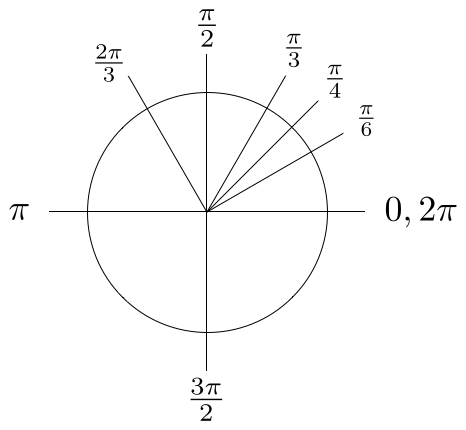But if we use $\large\tau$ all our problems vanish, and everything makes sense again. $\dfrac{1}{12}$ of the unit circle is $\dfrac{\large\tau}{\normalsize 12}$ radians, as shown in the picture below.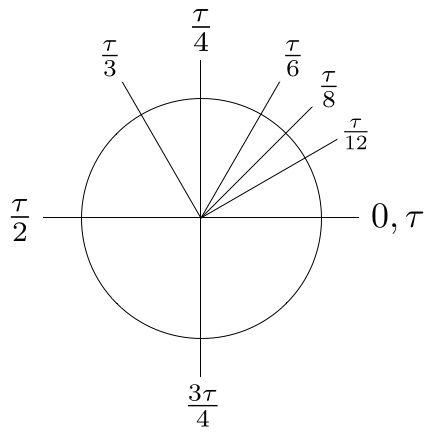This really shows that $\large\tau$ might actually be better to use than $\pi$. Yet, we all have our weaknesses, so let's look at the Disadvantages of $\large\tau$ next.

$\hspace{1px}$

## Disadvantages of $\large\tau$

$\large \text{Coming Soon!}$

$\hspace{1px}$

## $\large\tau$(Tau) vs $\pi$(Pi) Conflict

The Pi vs Tau Conflict is a very long conflict between people who love $\pi$ and people who love $\large\tau$.

It is a battle to find which of the $2$ irrational numbers is better and makes our calculations easier.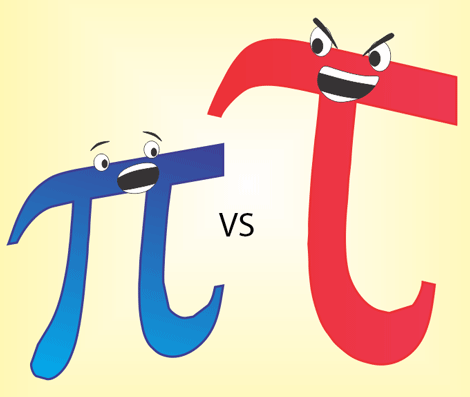An illustration of the epic battle :)

The basic arguments given by both teams were as follows -

$\hspace{1px}$

$\large \underline{\text{Team Tau against }\pi}$

$\pi = \dfrac{\text{Circumference}}{\text{Diameter}}$ while $\large\tau = \normalsize \dfrac{\text{Circumference}}{\text{Radius}}$

A circle is usually defined by its radius and the radius is something that mathematicians are generally more interested in than the diameter.

The formula for a circle's circumference is also simplified with $\large\tau$

$C = \large\tau\normalsize r$

$\large \tau$ Radians is equal to $360^{\circ}$, while $\pi$ Radians is equal to $180^{\circ}$. This value of Pi makes it a bit confusing in dealing with Trigonometry using Unit Circles, but Tau makes it look like easy as pie (pun intended)

$\hspace{1px}$

$\large \underline{\text{Team Pi against }\large \tau}$

Pi has been around since a long time. The number Tau also has its flaws. For example - The formula for the area of a circle is made even more complex by using $\large\tau$

$A = \pi r^{2} = \dfrac{\large\tau\normalsize r^{2}}{2}$

Also, redefining the circle constant differently and replacing it will destroy the beautiful Euler Identity - $e^{i\pi} + 1 = 0$

$\hspace{1px}$

This conflict is as long as the amount of digits in Pi and Tau. I will not take sides and either number isn't necessarily better. This is only a fragment of the conflict. This conflict has been around for a few years and hasn't officially ended yet.

$\hspace{1px}$

$\hspace{1px}$

## Related Wikis on Brilliant

$\hspace{1px}$

## Try some problems related to $\pi$ and $\large \tau$

$\hspace{1px}$

## More Digits

These are the first $100$ digits of Tau, but if you want more, go here -

$6.2831853071795864769252867665590057683943387987502116419498891846156328125724179972560696506842341359 \ldots$

$\hspace{1px}$

## Rate this Wiki

$\hspace{1px}$

## Next Update

$\large \text{Disadvantages of Tau - Due 21st to 25th Sep}$

Image Credits - Thanks to https://www.wikipedia.org/, for the 'Visualising Pi' image, the 'capital and small tau' image and the 'Pi vs Tau' image and to https://tauday.com/tau-manifesto for the Unit Circle images in 'Advantages of Tau'.

Cite as : Tau, Brilliant.org. Retrieved on some date and some time, from https://brilliant.org/discussions/thread/tau/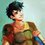Note by Percy Jackson
1 month, 1 week ago

This discussion board is a place to discuss our Daily Challenges and the math and science related to those challenges. Explanations are more than just a solution — they should explain the steps and thinking strategies that you used to obtain the solution. Comments should further the discussion of math and science.

When posting on Brilliant:

• Use the emojis to react to an explanation, whether you're congratulating a job well done , or just really confused .
• Ask specific questions about the challenge or the steps in somebody's explanation. Well-posed questions can add a lot to the discussion, but posting "I don't understand!" doesn't help anyone.
• Try to contribute something new to the discussion, whether it is an extension, generalization or other idea related to the challenge.

MarkdownAppears as
*italics* or _italics_ italics
**bold** or __bold__ bold
- bulleted- list
• bulleted
• list
1. numbered2. list
1. numbered
2. list
Note: you must add a full line of space before and after lists for them to show up correctly
paragraph 1paragraph 2

paragraph 1

paragraph 2

[example link](https://brilliant.org)example link
> This is a quote
This is a quote
    # I indented these lines
# 4 spaces, and now they show
# up as a code block.

print "hello world"
# I indented these lines
# 4 spaces, and now they show
# up as a code block.

print "hello world"
MathAppears as
Remember to wrap math in $$ ... $$ or $ ... $ to ensure proper formatting.
2 \times 3 $2 \times 3$
2^{34} $2^{34}$
a_{i-1} $a_{i-1}$
\frac{2}{3} $\frac{2}{3}$
\sqrt{2} $\sqrt{2}$
\sum_{i=1}^3 $\sum_{i=1}^3$
\sin \theta $\sin \theta$
\boxed{123} $\boxed{123}$

Sort by:

$\hspace{-50px}\color{grey}\\[-22px]\tiny\textsf{EXAMPLE}$
Sample Text

- 1 month, 1 week ago

How? Please comment this in Latex Club @Páll Márton

- 1 month, 1 week ago

so tau is double the value of pi even though the symbol is half of pi interesting

- 1 month, 1 week ago

Yes, a fun fact @Nathan Soliman :)

- 1 month, 1 week ago

what do we use tau to calculate

- 1 month, 1 week ago

Trigonometry graphs and functions that require unit circle method, because tau radians is 360 degrees :)

- 1 month, 1 week ago

- 1 week, 3 days ago

I've completed Advantages of Tau. Only Disadvantages left. It'll be done by next week :)

- 1 week, 1 day ago

@Frisk Dreemurr - I'm making a wiki for fun :)

How is it?

- 1 month, 1 week ago

- 1 month, 1 week ago

Nice @Percy Jackson, I never noticed any note on the debate of Tau and Pi until today :)

- 1 month, 1 week ago

Thanks, the debate is actually a pretty big thing :)

- 1 month, 1 week ago

@Frisk Dreemurr - I added a rating system LOL :)

- 1 month, 1 week ago

does it have a number count

- 1 month, 1 week ago

What? @Nathan Soliman

- 1 month, 1 week ago

the rating system

- 1 month, 1 week ago

No, its just for fun :)

- 1 month, 1 week ago

do you want to hear something stanley hudson said?

- 1 month, 1 week ago

@Páll Márton - I was looking at your formatting guide while making this, its really useful, thanks :)

- 1 month, 1 week ago

BTW go to my feed to see my latest note on buttons. You can add that to your guide @Páll Márton

- 1 month, 1 week ago

@Yajat Shamji - Look at this :)

- 1 month, 1 week ago

I really like it. However, I am impartial to both $\pi$ and $\tau$ so I am neutral in this war/conflict.

- 1 month, 1 week ago

I wrote that I'm neutral but I'm actually a Tauist LOL. Glad you like it :)

- 1 month, 1 week ago

@Yajat Shamji - There is an astronomy point in 'More About Tau' :)

- 1 month, 1 week ago

Thanks.

P.S. $5$ stars!

P.S.S. I challenge you to find geometric sums and limits involving $\pi$ that result in $\tau$ and geometric sums and limits involving $\tau$ that result in $\pi$.

- 1 month, 1 week ago

I'll try :) @Yajat Shamji

P.S Thanks you for 5 stars!

- 1 month, 1 week ago

Done @Yajat Shamji, I added a few integral formula's that I could think of. I still don't have a limit though, I'll work on that :)

- 1 week, 1 day ago

You know I'll make one...

- 1 week, 1 day ago

I found some (but the result's $\pi$ so you'll need to multiply the entire limit by $2$):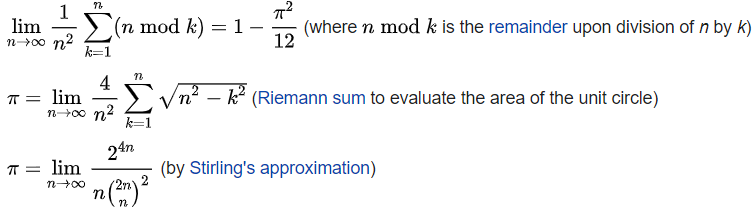- 1 week, 1 day ago

I need something where I don't need to multiply actually. There are many Pi limits - Pi limits

- 1 week, 1 day ago

@Yajat Shamji - I added a limit formula that doesn't need me to multiply the limit by 2 and do some crazy math, but I don't know its name.

- 1 week, 1 day ago

no, the beautiful Euler identity would not disappear, since e^i tau - 1 = 0 (but I am a pie fan)

- 1 week, 2 days ago

Its what they argue, its the truth. It may not be correct, but its an arguement

- 1 week, 2 days ago